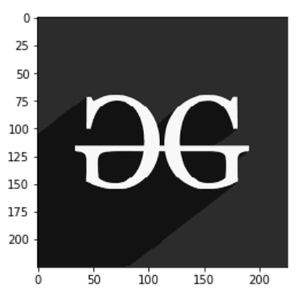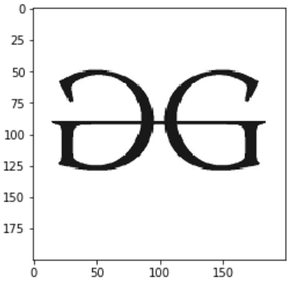GFG App
Open AppBrowser
Continue

# How to Display an Image in Grayscale in Matplotlib?

In this article, we are going to depict images using the Matplotlib module in grayscale representation using PIL, i.e. image representation using two colors only i.e. black and white.

Syntax: matplotlib.pyplot.imshow(X, cmap=None)

## Displaying Grayscale image

Displaying Grayscale image, store the image path here let’s say it fname. Now open the image using PIL image method and convert it to L mode If you have an L mode image, that means it is a single-channel image – normally interpreted as grayscale. It only stores a grayscale, not color. Plotting the image as cmap = ‘gray’ converts the colors. All the work is done you can now see your image.

## Python3

 `# storing image path` `fname ``=` `r``'g4g.png'`   `# opening image using pil` `image ``=` `Image.``open``(fname).convert(``"L"``)`   `# mapping image to gray scale` `plt.imshow(image, cmap``=``'gray'``)` `plt.show()`

Output:## Python3

 `# importing libraries.` `import` `numpy as np` `import` `matplotlib.pyplot as plt` `from` `PIL ``import` `Image`     `# storing image path` `fname ``=` `r``'gfg.png'`   `# opening image using pil` `image ``=` `Image.``open``(fname).convert(``"L"``)`   `# mapping image to gray scale` `plt.imshow(image, cmap``=``'gray'``)` `plt.show()`

Output:Image used## Python3

 `# importing libraries.` `import` `numpy as np` `import` `matplotlib.pyplot as plt` `from` `PIL ``import` `Image`     `# storing image path` `fname ``=` `r``'geeks.png'`   `# opening image using pil` `image ``=` `Image.``open``(fname).convert(``"L"``)`   `# mapping image to gray scale` `plt.imshow(image, cmap``=``'gray'``)` `plt.show()`

Output:Image UsedMy Personal Notes arrow_drop_up